Scientific and technical journal

### 2009### Contacts

F. Sсorina Gomel State University
Sovetskaya Str., 104, 246019, Gomel,
Republic of Belarus

Phones:
+375 (232) 51-00-77
+375 (232) 51-03-21
+375 (29) 678-62-50

E-mail:
pfmt*gsu.by

Website:
http://pfmt.gsu.by

## MATERIALS: № 1 (38) 2019

PHYSICS

#### Avlasevich N.T., Lyalikov A.M. Holographic interferometry of dynamic periodic structures in real time

A method of recording and subsequent restoration of a hologram of transmissive periodic structures by light waves diffracted directly on the structure under study is proposed. It is shown that the use of such a reference hologram allows reconstructing the interference patterns that reflect the behavior of the structure parameters in time. The experimental results of testing the proposed method in the study of the dynamics of two-dimensional periodic structures in real time are presented.

Keywords: dynamic periodic structure, light wave, hologram, holographic interferometry, visualization of defect, interference pattern.

N.T. Avlasevich – Y. Kupala Grodno State University

A.M. Lyalikov – Y. Kupala Grodno State University

#### Andreev V.V., Maksimenko N.V., Deryuzhkova О.М. Speenless particle motion equations in the electromagnetic field considering dipole polarizability

Using the relativistic Lagrange – Euler equations, the equations of motion for a charged structural particle of a spin 0 in the electromagnetic field are obtained. The Lagrangian of the electromagnetic field interaction with a structural spinless particle contains dipole polarizabilities that are consistent with polarizabilities which are included in the amplitude of Compton scattering on a spinless particle. This Lagrangian and the amplitude are obtained based on the gauge-invariant approach and on the solution of electrodynamic equations using the Green function method.

Keywords: charged structural spinless particle, dipole polarizabilities, Lagrangian, amplitude of Compton scattering.

V.V. Andreev – Faculty of Physics and Information Technology, F. Scorina Gomel State University

N.V. Maksimenko – Faculty of Physics and Information Technology, F. Scorina Gomel State University

О.М. Deryuzhkova – Faculty of Physics and Information Technology, F. Scorina Gomel State University

#### Girgel S.S. Circular 3D Kummer - Gauss beams with the continuous angular index

Analytical expressions in the closed shape for circular 3D Kummer - Gauss beams (cK-G) with the continuous angular coefficient m are offered and analyzed. Physical restrictions on possible values of the free parameters of such beams are formulated. Pictorial modeling of beams cK-G is fulfilled and shown that the continuous values of an angular coefficient m and complex values of the free parameter v are physically possible.

Keywords:

S.S. Girgel – Faculty of Physics and Information Technology, F. Scorina Gomel State University

#### Naunyka V.N., Amanova M.A., Makarevich A.V., Yudzitski U.V. Effect of optical activity and absorption on contradirectional two-wave interaction in Bi12TiO20 crystal

The dependence of relative intensity of the subject light wave on azimuths of polarization of the pump and subject waves at mutual diffraction by reflection holographic grating formed in Bi12TiO20 crystal is investigated. It is shown that combined effect of optical activity and absorption leads to changing of azimuths of polarization of the light waves for which maximal values of relative intensity of the subject wave are achieved. The rule of how to choose the input azimuths of polarization for achieving maximal gain of the subject wave by contradirectional two-wave interaction by reflection holographic grating in optical active crystal is established.

Keywords: reflection hologram, two-wave interaction, relative intensity of the subject wave, azimuth of polarization, optical activity, absorption.

V.N. Naunyka – I.P. Shamyakin Mozyr State Pedagogical University

M.A. Amanova – I.P. Shamyakin Mozyr State Pedagogical University

A.V. Makarevich – I.P. Shamyakin Mozyr State Pedagogical University

U.V. Yudzitski – Belarusian State University of Informatics and Radioelectronics

MATHEMATICS

#### Borodich R.V. On intersection of abnormal subgroups that don`t contain a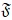-residual

The structure of a subgroup equal to the intersection of maximal A-admissible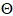-subgroups not containing theKeywords: finite group, abnormal subgroup,-residual.

R.V. Borodich – Faculty of Mathematics and Programming Technologies, F. Scorina Gomel State University

#### Gal'mak A.M. On non-n-semiabelianism polyadic groupoids of special class

The permutability of the elements in polyadic groupoids with polyadic operation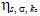that is defined on Cartesian power of Ak n-ary groupoid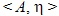substitution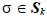and n-ary operation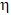are considered. The main result of the article is the theorem in which sufficient conditions of non-n-semiabelianism of l-ary (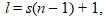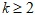) groupoid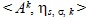are formulated. Numerous conse-quences of this theorem are given. In particular, it was found that if substitution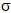satisfies the conditions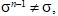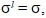n-ary grouphas no less than two elements, then polyadic groupoidis a non-n-semiabelian polyadic group.

Keywords: polyadic operation, n-ary groupoid, abelianism, semiabelianism, neutral sequence.

A.M. Gal'mak – Mogilev State University of Food Technologies

#### Hundzina М.А. Determination of the initial direction of cracks growth at the moment of movement

A technique for determining the initial direction of the growth of a straight crack in a plate of elastic material with a load, applied at the boundaries of the plate in the plane strain conditions, is considered. In the paper analytical representations for the stress tensor components are given. The formulation of the problem and the sequence of steps for finding the value of the angle, at which subcritical crack growth begins, are described. The obtained theoretical dependences by definition of the characteristics of the limiting state of the construction allow making a motivated choice of geometric parameters with the strength properties of the material.

Keywords: crack, initial direction of crack growth, stress intensity, material strength.

М.А. Hundzina – Belarusian National Technical University, Minsk

#### Zelenaya A.S. Cylindrical bending of elastoplastic rectangular three-layer plate with compressible filler in temperature field

Cylindrical bending of asymmetrical in thickness elastic-thermoplastic three-layer plates with a compressible filler under the action of loads and temperature was investigated. The kinematic hypotheses are based on the hypothesis of a broken line: the Kirchhoff hypotheses are accepted for the outer layers, the deformed normal remains rectilinear in a rigid compressible filler. A system of equilibrium equations and its analytical solution in displacements are obtained. Numerical realization of solutions for a three-layer metal-polymer plate is carried out.

Keywords: thermoplasticity, three-layered rectangular plate, cylindrical bending.

A.S. Zelenaya – Belarusian State University of Transport, Gomel

#### Kamornikov S.F., Shemetkova O.L. On some characterization of general Frattini subgroup of finite soluble group

Let G be a finite soluble group,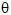be a regular subgroup m-functor, and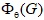be the intersection of all maximal-subgroups of G. Let n be the length of a G-series of the group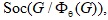and k be the number of central G-chief factors of this series. We prove that in this case G contains 4n-3k maximal-subgroups whose intersection is.

Keywords: finite soluble group, maximal subgroup, Frattini-subgroup.

S.F. Kamornikov – F. Scorina Gomel State University

O.L. Shemetkova – Plekhanov Russian University of Economics, Moscow

#### Mirotin A.R., Kovaliova I.S. The Markov - Stieltjes transform of measures and discrete time systems

А class of discrete time filters (systems) with frequency characteristics that are functions of Markov - Stieltjes type is considered. The description of these filters in terms of their system functions and impulse responses is announced. In particular, it is noted that this class contains all filters with completely monotonic impulse responses. The properties of stationarity, causality, stability and reversibility of the corresponding systems are described.

Keywords: Markov - Stieltjes transform, filter, frequency characteristic, system function, stationary state, causality, stable system, invertible system.

A.R. Mirotin – Faculty of Mathematics and Programming Technologies, F. Scorina Gomel State University

I.S. Kovaliova – Faculty of Mathematics and Programming Technologies, F. Scorina Gomel State University

#### Monakhov V.S., Khadanovich D.A. On the solvability of a finite group with a pair of non-conjugate subgroups of primary indices II

The solvability of a finite group G with two non-conjugate maximal subgroups A and B that satisfy the following requirements has been proved: subgroups A and B have primary indices in G; all proper subgroups of A and B are 2-nilpotent. In addition, if G is S4-free and the indices of the subgroups A and B are coprime, then the 2-nilpotency of the subgroups A and B can be replaced by their solvability.

Keywords: finite group, solvable group, maximal subgroup, 2-nilpotent subgroup, non-conjugate subgroups, primary index.

V.S. Monakhov – Faculty of Mathematics and Programming Technologies, F. Scorina Gomel State University

D.A. Khadanovich – Faculty of Mathematics and Programming Technologies, F. Scorina Gomel State University

#### Ruzhitskaya E.A., Karaseva G.L. Application of the problem of speed for realizing of a given motion of a dynamic system

The problem of the given motions realization by dynamic systems is investigated. Using the optimal control methods current values of limited optimal feedbacks are calculated with the help of solution of special auxiliary optimal control problems - speed problems. The results are illustrated by the example of the second order dynamical system, realizing a limited cycle.

Keywords: stabilization, optimal feedback, dynamic system, speed problem, optimal control problem, problem of realizing given motions.

E.A. Ruzhitskaya – Faculty of Mathematics and Programming Technologies, F. Scorina Gomel State University

G.L. Karaseva – Faculty of Mathematics and Programming Technologies, F. Scorina Gomel State University

#### Trofimuk A.A., Zubei E.V. On the permutability of a Sylow subgroup with Schmidt subgroups of odd order

A finite non-nilpotent group G is called a Schmidt group if every proper subgroup of G is nilpotent. In this paper the non-abelian composition factors of a group in which a Sylow subgroup is permutable with Schmidt subgroups of odd order is determined.

Keywords: finite group, solvable group, Schmidt subgroup, Sylow subgroup, permutable subgroups.

A.A. Trofimuk – Faculty of Mathematics and Programming Technologies, F. Scorina Gomel State University

E.V. Zubei – Faculty of Mathematics and Programming Technologies, F. Scorina Gomel State University

#### Zhang B., Chen Y., Martynov I.P. The first integrals and rational solutions of differential equations with a moving singular line

The nonlinear autonomous higher-order differential equations with a moving singular line are studied. The first integrals of the Chazy equation and some other equations with a moving singular line are obtained. Nonlinear differential equations, for which general solutions are rational solutions of equations with a moving singular line are obtained. It is shown that with the help of Backlund transformations, rational solutions of equations with a movable singular line can be transformed into each other. Nonlinear differential equations of the second or third degree with respect to the highest derivative are obtained, for which these rational solutions are general solutions.

Keywords: differential equations, resonances, rational solutions, first integrals, Backlund transform, movable singular line.

Zhang Binbin – post-graduate student of the Department of Mathematical Analysis, Differential Equations and Algebra, Yanka Kupala Grodno State University

Chen Yang – post-graduate student of the Department of Mathematical Analysis, Differential Equations and Algebra, Yanka Kupala Grodno State University

Martynov Ivan Platonovich – doctor of physical and mathematical sciences, professor of the Department of Mathematical Analysis, Differential Equations and Algebra, Yanka Kupala Grodno State University

INFORMATION SCIENCE

#### Kurachkin A.V., Sadau V.S., Demidenko O.M. Optimizing the decision-making process in fuzzy logic medical expert systems using historical data

Two of the most popular approaches to building decision support systems are fuzzy inference systems and supervised machine learning systems. However, fuzzy inference systems are based solely on expert decision-making process formalization and don’t take historical data into account, while machine learning systems infer certain statistical dependencies based solely on historical data, and those dependencies are very hard to formally analyze reason about from an expert point of view. The possible ways of combining these two approaches - building and optimizing medical fuzzy inference systems based on historical data are considered.

Keywords: expert systems, medical expert systems, fuzzy logic, machine learning.

A.V. Kurachkin – Belarusian State University, Minsk

V.S. Sadau – Belarusian State University, Minsk

O.M. Demidenko – F. Scorina Gomel State University

#### Mozharovsky V.V., Kuzmenkov D.S., Golubeva E.A. Realization of the solution of viscoelasticity’ problems for a pipe from a functional gradient material

The stress-strain state of a viscoelastic tube made of inhomogeneous materials under the action of internal pressure is determined. The main dependences for the calculation of pipes made of heterogeneous materials are presented. On the basis of the received formulas for finding deformations the computer program of calculation of deformations in a pipe changing in time is created. The implementation of calculations of the stress-strain state in the pipe and the parameters of creep and relaxation nuclei is presented.

Keywords: viscoelasticity, pipe, functionally graded material, strain.

V.V. Mozharovsky – Faculty of Mathematics and Programming Technologies, F. Scorina Gomel State University

D.S. Kuzmenkov – Faculty of Mathematics and Programming Technologies, F. Scorina Gomel State University

E.A. Golubeva – Faculty of Mathematics and Programming Technologies, F. Scorina Gomel State University

#### Chechat P.L., Varuyeu A.V., Liauchuk A.A. Design of simulation experiments for comparing elevators control strategies in a high-rise building

The design of simulation experiments for comparing elevators control strategies in a high-rise building is considered. Model parameters are control algorithms, not the values of individual parameters. A design pattern is proposed for the simulator that allows both trivially to add control strategies and create new control strategies based on already developed ones.

Keywords: elevators control strategy, simulator, simulation experiment, comparison of alternatives.

P.L. Chechet – Faculty of Physics and Information Technology, F. Scorina Gomel State University

A.V. Varuyeu – Faculty of Physics and Information Technology, F. Scorina Gomel State University

A.A. Liauchuk – Belarusian Trade and Economics University of Consumer Cooperatives, Gomel